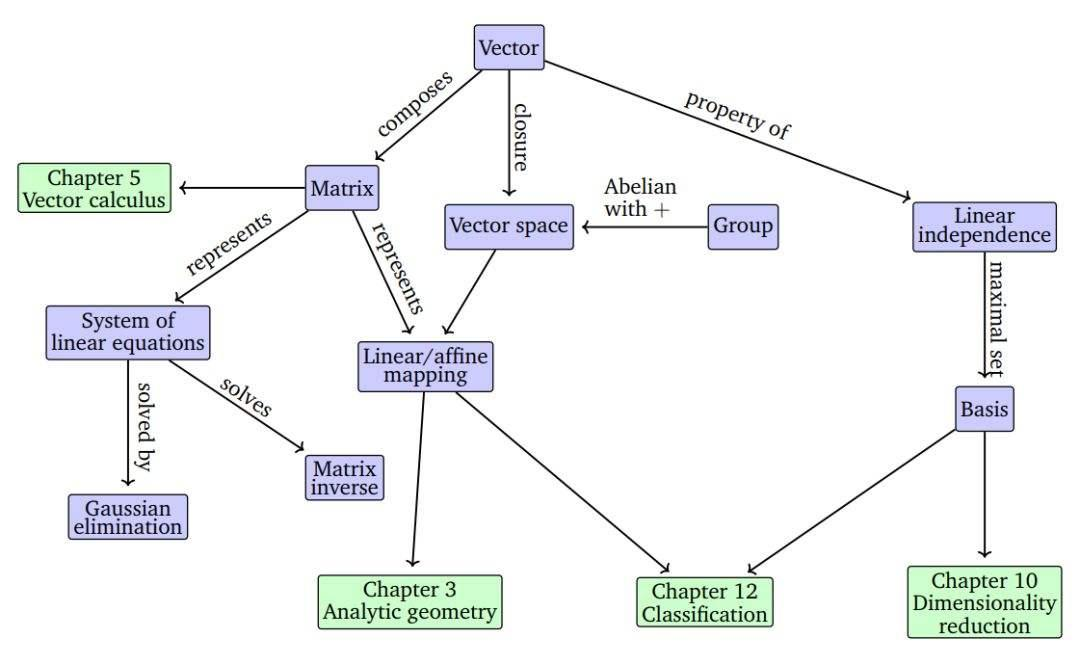## (1)首先我们简单介绍一下什么是webshell：

webshell，是一种基于互联网web程序以及web服务器而存在的一种后门形式，主要通过网页脚本程序和服务器容器所支持的后端程序，在web服务器及其中间件中进行运行。

Webshell通常针对web开放端口，并使用脚本语言编写一般一个webshell主程序整体是一个正向的链接，可以被攻击者主动访问。在攻击链模型中，一次针对webshell攻击的过程主要分为以下步骤：踩点，组装，投送，攻击，植入，控制以及行为。

## (2)上代码

``````import numpy as np
import pandas as pd
import matplotlib.pyplot as plt
import sklearn
import os
%matplotlib inline
import warnings

warnings.filterwarnings('ignore')
``````

php-webshell文件夹中存放着笔者收集而来的大量webshell样本并将它们通过md5检验去重

``````files_webshell = os.listdir("/webshell/project/php-webshell/")
files_common = os.listdir("/webshell/project/php-common")``````

``````labels_webshell = []
labels_common = []
for i in range(0,len(files_webshell)):
labels_webshell.append(1)
for i in range(0,len(files_common)):
labels_common.append(0)``````

``````for i in range(0,len(files_webshell)):
files_webshell[i] = "/webshell/project/php-webshell/" + files_webshell[i]
for i in range(0,len(files_common)):
files_common[i] = "/webshell/project/php-common/" + files_common[i]

files = files_webshell + files_common
labels = labels_webshell + labels_common

``````def getfilelen(x):
length = 0
with open(x,'r',encoding='ISO-8859-1') as f:
for i in content:
length = length + len(i)
f.close()
return length

df['len'] = df['file'].map(lambda x:getfilelen(x)).astype(int)``````

``````import seaborn as sns
plt.style.use('seaborn')
sns.set(font_scale=2)
pd.set_option('display.max_columns', 500)

G = -ΣLog(P(Xi)) * P(Xi)

``````def getfileshan(x):
length = 0
word = {}
p = 0
sum = 0
with open(x,'r',encoding='ISO-8859-1') as f:
for i in content:
for j in i:
if j != '\n' and j != ' ':
if j not in word.keys():
word[j] = 1
else:
word[j] = word[j] + 1
else:
pass
f.close()
for i in word.keys():
sum = sum + word[i]
for i in word.keys():
p = p - float(word[i])/sum * math.log(float(word[i])/sum,2)
return p

df['shan'] = df['file'].map(lambda x:getfileshan(x)).astype(float)``````

（1）文件，目录操作类函数

（2）解码编码类函数

（3）文件压缩类函数

（4）字符编码转换类函数

（5）字符替换类函数

（6）动态函数类等其他敏感不常见操作

``````import re
def getfilefunc(x):
content = ''
content_list = []
with open(x,'r',encoding='ISO-8859-1') as f:
for i in c:
content = content + i.strip('\n')
f.close()
content_list = re.split(r'\(|\)|\[|\]|\{|\}|\s|\.',content)
max_length = 0
for i in content_list:
if len(i) > max_length:
max_length = len(i)
else:
pass
#print(content_list)
count_exec = 0
count_file = 0
count_zip = 0
count_code = 0
count_chr = 0
count_re = 0
count_other = 0
for i in content_list:
if 'assert' in i or 'system' in i or 'eval' in i or 'cmd_shell' in i or 'shell_exec' in i:
count_exec = count_exec + 1
if 'file_get_contents' in i or 'fopen' in i or 'fwrite' in i or 'readdir' in i or 'scandir' in i or 'opendir' in i or 'curl' in i:
count_file = count_file + 1
if 'base64_encode' in i or 'base64_decode' in i:
count_code = count_code + 1
if 'gzcompress' in i or 'gzuncompress' in i or 'gzinflate' in i or 'gzdecode' in i:
count_zip = count_zip + 1
if 'chr' in i or 'ord' in i:
count_chr + count_chr + 1
if 'str_replace' in i or 'preg_replace' in i or 'substr' in i:
count_re = count_re + 1
if 'create_function' in i or 'pack' in i:
count_other = count_other + 1
#print(x)
return (max_length,count_exec,count_file,count_zip,count_code,count_chr,count_re,count_other)``````

``````df['maxlen'] = df['func'].map(lambda x:x)
df['exec'] = df['func'].map(lambda x:x)
df['file'] = df['func'].map(lambda x:x)
df['zip'] = df['func'].map(lambda x:x)
df['code'] = df['func'].map(lambda x:x)
df['chr'] = df['func'].map(lambda x:x)
df['re'] = df['func'].map(lambda x:x)
df['other'] = df['func'].map(lambda x:x)``````

``````from sklearn import preprocessing
from sklearn.utils import shuffle
from sklearn.svm import SVC
from sklearn.linear_model.logistic import LogisticRegression``````

``````scaler = preprocessing.StandardScaler()

len_scale_param = scaler.fit(df['len'].values.reshape(-1,1))
df['len_scaled'] = scaler.fit_transform(df['len'].values.reshape(-1,1),len_scale_param)``````

``````train_pre = df.filter(regex = 'label|len_scaled|shan_sclaed|maxlen_sclaed|exec_sclaed|zip_sclaed|code_sclaed')
train_pre = shuffle(train_pre)``````

``train_pre =train_pre.as_matrix()``

``````y_train = train_pre[0:7000,0]
x_train = train_pre[0:7000,1:]
y_test = train_pre[7000:,0]
x_test = train_pre[7000:,1:]``````

``````print ('now training')
lr = LogisticRegression().fit(x_train,y_train)
print ('training finished')
model = lr.predict(x_test)

from sklearn import metrics
from sklearn.metrics import accuracy_score
accuracy_score(model,y_test)

OUT:0.8010139416983524``````

xgboost时一种提升方法，他和lgbm是目前算法竞赛重用的最广泛的机器学习模型（

model = xgb.XGBClassifier(max_depth=3, n_estimators=10000, learn_rate=0.01)

``````params = {
'booster': 'gbtree',
'num_class': 2,               # 类别数，与 multisoftmax 并用
'gamma': 0.1,                  # 用于控制是否后剪枝的参数,越大越保守，一般0.1、0.2这样子。
'max_depth': 12,               # 构建树的深度，越大越容易过拟合
'lambda': 2,                   # 控制模型复杂度的权重值的L2正则化项参数，参数越大，模型越不容易过拟合。
'subsample': 0.7,              # 随机采样训练样本
'colsample_bytree': 0.7,       # 生成树时进行的列采样
'min_child_weight': 3,
'silent': 1,                   # 设置成1则没有运行信息输出，最好是设置为0.
'eta': 0.007,
'seed': 1000,
}

params['eval_metric'] = 'error'
num_round = 200
dtest = xgb.DMatrix( x_test, label=y_test)
evallist  = [(dtest,'test'), (dtrain,'train')]
model = xgb.XGBClassifier(max_depth=3, n_estimators=10000, learn_rate=0.01)
model.fit(x_train, y_train)
test_score = model.score(x_test, y_test)``````

``````test_score
OUT:0.9467680608365019``````